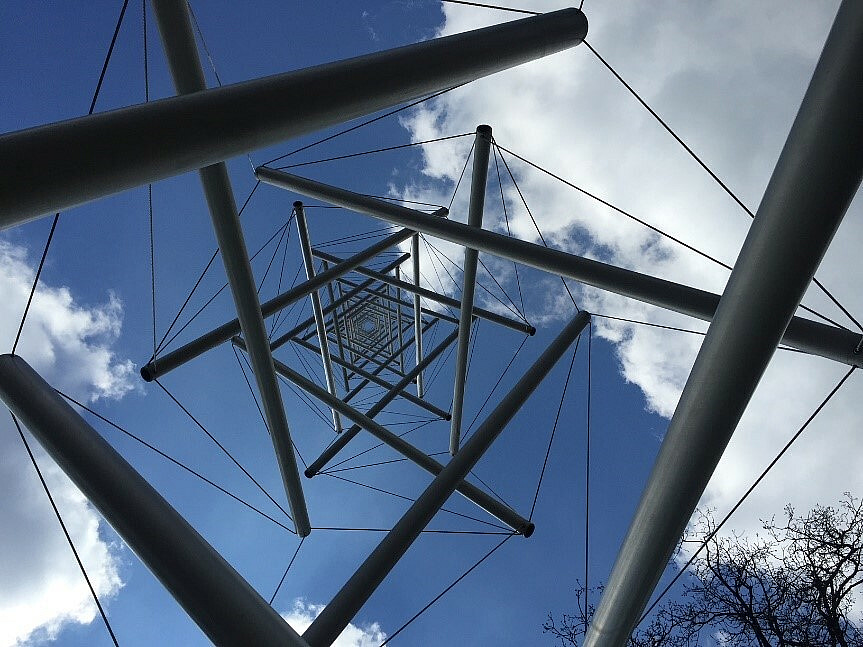# Accelerating topology optimization

Introducing Deep Learning techniques to topological optimization reduces computational time and could expand its application scope.Topology optimization is a method to optimize the material layout inside a given domain and has wide applications from fluid mechanics to infrastructure design, and from heat transfer to aerospace. However, current optimization approaches have a high computational cost limiting their application to small, simple geometries.  Now, research published in Nature Communications reports an approach with reduced computational load enabling application of topological optimization to more complex structures.

Finite Element Method (FEM) is a numerical approach often used in topology optimization, allowing the user to approximate a solution by discretizing a large domain into small elements. Like other optimizers, FE-optimizations can be roughly categorized into gradient-based optimizers and non-gradient optimizers depending on whether  a gradient (or derivative of the function) is required for FE evaluation.   The demands of equation gradient information constrains its application and implementation to simple problems.  On the other hand, non-gradient topology optimizers are capable of computing complex problems and geometries but become extremely expensive when scaling to large systems. 

Machine learning is an efficient surrogate model for expensive calculations to reduce the computational cost.  However, the benefits of applying machine learning to topology optimization are still limited by the searching algorithms and the coverage of training datasets, with resulting application only in small-scale and low-dimensional problems. For example the required training data points increase exponentially in higher dimensions or the algorithms are incapable of finding minima.

Wei Lu and his group from University of Michigan, Ann Arbor proposed a Self-directed Online Learning Optimization (SOLO) method, embedding deep learning techniques, which dramatically reduced the computational time compared to all other state-of-art methods.

In topology optimization, the calculation of the objective function (the function that represents the quantity (energy, pressure etc.) being evaluated for best performance) is time-consuming using FEM. The SOLO algorithm reduces the number of FEM calculations by introducing a surrogate Deep Neural Network (DNN), an artificial network that can model complex non-linear relationships to approximate the objective function. DNN is trained using data dynamically generated by FEM and focuses on the performance near the optimum reducing the size of the training dataset.

After FEM calculation of a small set of designed input data, the SOLO method begins. First, the economic surrogate DNN model is used to approximate the objective function. Then, the global optimal value is calculated from the DNN using an heuristic algorithm - an algorithm that searches the entire domain. Finally, to achieve higher resolution, the optimal data is modified by adding a small perturbation- replacing, exchanging several data with random vectors or adding a filter in the obtained optimal data, to retrain the DNN. The loop will continue until convergence appears, when the value does not change anymore.

To test the performance of their SOLO method, the researchers applied the approach to four application directions: maximizing the stiffness of a solid structure,  minimizing fluidic pressure loss in a tunnel system, minimizing charging time of a heat storage system and finally the design of truss structure for minimized weight (often used for antenna tower design, such as that shown in the figure above).

The researchers benchmarked to different optimizers, and found that SOLO outperformed all the other tested algorithms, showing faster convergence to the global optimum.  For example, compared to a heuristic algorithm for truss optimization, SOLO demonstrated higher computational efficiency, reducing the number of FEM computations by over two orders of magnitude.

Topology optimization has developed tremendously since its introduction in 1988 and provides solutions to numerous engineering problems . With contributions from deep learning techniques, the proposed SOLO algorithm enables faster optimizations to solve complex and large scale problems, thus expanding the potential application scope of topology optimization.

References

 Sigmund, O. On the usefulness of non-gradient approaches in topology optimization. Struct Multidisc Optim 43, 589–596 (2011). DOI: https://doi.org/10.1007/s00158-011-0638-7

 Qian, C., Ye, W. Accelerating gradient-based topology optimization design with dual-model artificial neural networks. Struct Multidisc Optim 63, 1687–1707 (2021). DOI: https://doi.org/10.1007/s00158-020-02770-6

The original article can be found here:

Deng, C., Wang, Y., Qin, C. et al. Self-directed online machine learning for topology optimization. Nat Commun 13, 388 (2022). https://doi.org/10.1038/s41467-021-27713-7